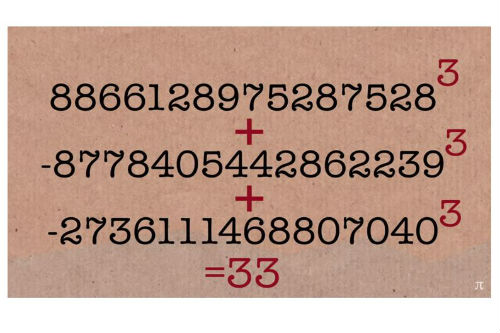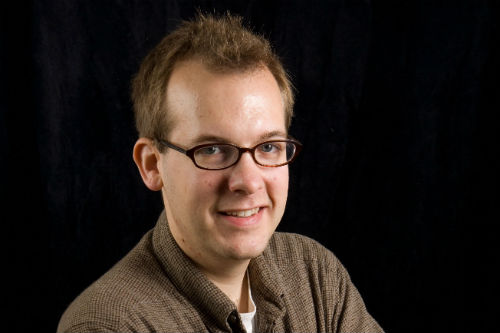# Bristol mathematician cracks Diophantine puzzleDr Andrew Booker University of Bristol

Press release issued: 1 April 2019

A mathematician from the University of Bristol has found a solution to part of a 64-year old mathematical problem – expressing the number 33 as the sum of three cubes.

Since the 1950s, mathematicians have wondered if all whole numbers could be expressed as the sum of three cubes; whether the equation k = x³+ y³+ z³ always has a solution.

The puzzle is a Diophantine equation in the field of number theory, and forms part of one of the most mysterious and wickedly hard problems in mathematics. We still don’t know the answer.

As computing power has increased more of these solutions were identified, as well as a group that we know have no solutions; those that leave remainder 4 or 5 when divided by 9. Until recently there were just two more unknown solutions under 100 remaining; 33 and 42.

Dr Andrew Booker, Reader of Pure Mathematics from the University’s School of Mathematics, has now discovered the solution for number 33: (8,866,128,975,287,528)³ + (–8,778,405,442,862,239)³ + (–2,736,111,468,807,040)³.

After watching a YouTube video where former Bristol mathematician Professor Tim Browning explained the problem, he was hooked.

“The video was called ‘The Uncracked Problem’”, he said. “That made me want to have a go!”

Dr Booker had expected to do a much more extensive search, but the computer identified a solution after a couple of weeks.

He said: “I had a pretty good guess that I’d find something for one of the numbers below 1000. But I didn’t know it was going to be the number 33.”

“We don’t know if the remaining numbers have infinitely many solutions, or how frequent those solutions are. It’s quite mysterious.”

Historically, the conjecture was that solutions didn’t exist for some of these numbers – that they were impossible to solve.

Mathematicians simply don’t know if they will ever be able to answer this for every number; it could be that the sum of three cubes problem is undecidable, or independent from the axioms of mathematics.

But each new discovery provides evidence for the modern conjecture that all eligible numbers have solutions.

Dr Booker said: “This one’s right at the boundary between what we know how to prove and what we suspect might be undecidable.”

The next and final unsolved number under 100 – 42 – has the appeal of being Douglas Adams’ answer to the meaning of life. Dr Booker is currently working with Andrew Sutherland from MIT to find it.

## Further information

Paper:

‘Cracking the problem with 33’ by A. Booker

Watch the videos on Numberphile which inspired the solution: The Uncracked Problem with 33 and 74 is cracked.